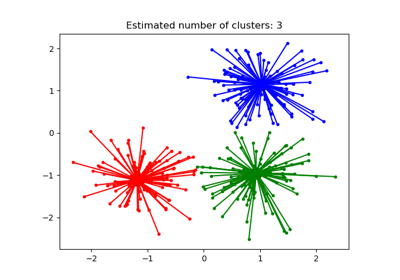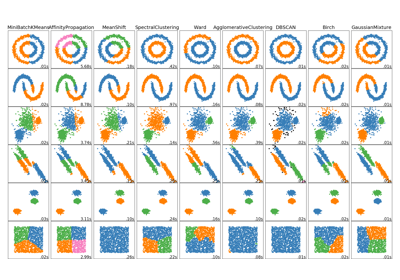# sklearn.cluster.AffinityPropagation¶

class sklearn.cluster.AffinityPropagation(damping=0.5, max_iter=200, convergence_iter=15, copy=True, preference=None, affinity='euclidean', verbose=False)[source]

Perform Affinity Propagation Clustering of data.

Read more in the User Guide.

Parameters: damping : float, optional, default: 0.5 Damping factor (between 0.5 and 1) is the extent to which the current value is maintained relative to incoming values (weighted 1 - damping). This in order to avoid numerical oscillations when updating these values (messages). max_iter : int, optional, default: 200 Maximum number of iterations. convergence_iter : int, optional, default: 15 Number of iterations with no change in the number of estimated clusters that stops the convergence. copy : boolean, optional, default: True Make a copy of input data. preference : array-like, shape (n_samples,) or float, optional Preferences for each point - points with larger values of preferences are more likely to be chosen as exemplars. The number of exemplars, ie of clusters, is influenced by the input preferences value. If the preferences are not passed as arguments, they will be set to the median of the input similarities. affinity : string, optional, default=euclidean Which affinity to use. At the moment precomputed and euclidean are supported. euclidean uses the negative squared euclidean distance between points. verbose : boolean, optional, default: False Whether to be verbose. cluster_centers_indices_ : array, shape (n_clusters,) Indices of cluster centers cluster_centers_ : array, shape (n_clusters, n_features) Cluster centers (if affinity != precomputed). labels_ : array, shape (n_samples,) Labels of each point affinity_matrix_ : array, shape (n_samples, n_samples) Stores the affinity matrix used in fit. n_iter_ : int Number of iterations taken to converge.

Notes

For an example, see examples/cluster/plot_affinity_propagation.py.

The algorithmic complexity of affinity propagation is quadratic in the number of points.

When fit does not converge, cluster_centers_ becomes an empty array and all training samples will be labelled as -1. In addition, predict will then label every sample as -1.

When all training samples have equal similarities and equal preferences, the assignment of cluster centers and labels depends on the preference. If the preference is smaller than the similarities, fit will result in a single cluster center and label 0 for every sample. Otherwise, every training sample becomes its own cluster center and is assigned a unique label.

References

Brendan J. Frey and Delbert Dueck, “Clustering by Passing Messages Between Data Points”, Science Feb. 2007

Examples

>>> from sklearn.cluster import AffinityPropagation
>>> import numpy as np
>>> X = np.array([[1, 2], [1, 4], [1, 0],
...               [4, 2], [4, 4], [4, 0]])
>>> clustering = AffinityPropagation().fit(X)
>>> clustering
AffinityPropagation(affinity='euclidean', convergence_iter=15, copy=True,
damping=0.5, max_iter=200, preference=None, verbose=False)
>>> clustering.labels_
array([0, 0, 0, 1, 1, 1])
>>> clustering.predict([[0, 0], [4, 4]])
array([0, 1])
>>> clustering.cluster_centers_
array([[1, 2],
[4, 2]])


Methods

 fit(X[, y]) Create affinity matrix from negative euclidean distances, then apply affinity propagation clustering. fit_predict(X[, y]) Performs clustering on X and returns cluster labels. get_params([deep]) Get parameters for this estimator. predict(X) Predict the closest cluster each sample in X belongs to. set_params(**params) Set the parameters of this estimator.
__init__(damping=0.5, max_iter=200, convergence_iter=15, copy=True, preference=None, affinity='euclidean', verbose=False)[source]
fit(X, y=None)[source]

Create affinity matrix from negative euclidean distances, then apply affinity propagation clustering.

Parameters: X : array-like, shape (n_samples, n_features) or (n_samples, n_samples) Data matrix or, if affinity is precomputed, matrix of similarities / affinities. y : Ignored
fit_predict(X, y=None)[source]

Performs clustering on X and returns cluster labels.

Parameters: X : ndarray, shape (n_samples, n_features) Input data. y : Ignored not used, present for API consistency by convention. labels : ndarray, shape (n_samples,) cluster labels
get_params(deep=True)[source]

Get parameters for this estimator.

Parameters: deep : boolean, optional If True, will return the parameters for this estimator and contained subobjects that are estimators. params : mapping of string to any Parameter names mapped to their values.
predict(X)[source]

Predict the closest cluster each sample in X belongs to.

Parameters: X : {array-like, sparse matrix}, shape (n_samples, n_features) New data to predict. labels : array, shape (n_samples,) Index of the cluster each sample belongs to.
set_params(**params)[source]

Set the parameters of this estimator.

The method works on simple estimators as well as on nested objects (such as pipelines). The latter have parameters of the form <component>__<parameter> so that it’s possible to update each component of a nested object.

Returns: self

## Examples using sklearn.cluster.AffinityPropagation¶Demo of affinity propagation clustering algorithmComparing different clustering algorithms on toy datasets